# Gram determinant

(diff) ← Older revision | Latest revision (diff) | Newer revision → (diff)

A determinant of the form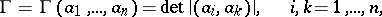where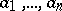are elements of a (pre-)Hilbert space and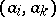are their scalar products. A Gram determinant is equal to the square of the-dimensional volume of the parallelotope constructed on.

A Gram determinant is the determinant of a non-negative Hermitian form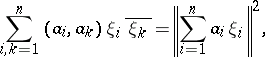which determines its basic properties:

1) A Gram determinant is non-negative, i.e.. The equality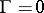is valid if and only if the vectors are linearly dependent. This property can be regarded as a generalization of the Cauchy inequality:or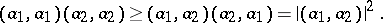In particular, a Gram determinant is equal to zero if any of its principal minors (which is also a Gram determinant) is zero.

2)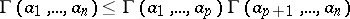, equality holding if and only if the subspacesandare orthogonal or if one of the determinants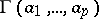,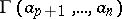is equal to zero. The geometrical meaning of this inequality is that the volume of the parallelotope is not larger than the product of the volumes of complementary faces. In particular,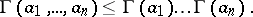3)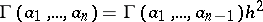, where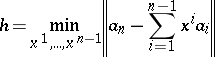is the distance from the element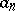to the subspace, i.e. the best quadratic approximation (cf. Best approximation) to the elementby polynomials of the type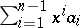.

Ifare-dimensional vectors,, thenGram determinants were introduced by J.P. Gram  and, independently, by K.A. Andreev  in the context of problems of expansion of functions into orthogonal series and the best quadratic approximation to functions.

The Gram determinant is used in many problems of linear algebra and function theory: studies of linear dependence of systems of vectors or functions, orthogonalization of systems of functions, construction of projections, and also in studies on the properties of systems of functions. See also Gram matrix.

The Gram determinant is a special case of determinants of the type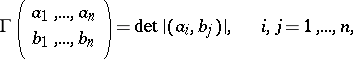which are Hermitian and bilinear with respect to vectors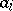and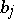. If allare of class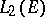, then the following formula is valid: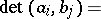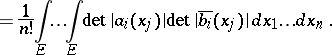How to Cite This Entry:
Gram determinant. Encyclopedia of Mathematics. URL: http://encyclopediaofmath.org/index.php?title=Gram_determinant&oldid=18442
This article was adapted from an original article by L.P. Kuptsov (originator), which appeared in Encyclopedia of Mathematics - ISBN 1402006098. See original article